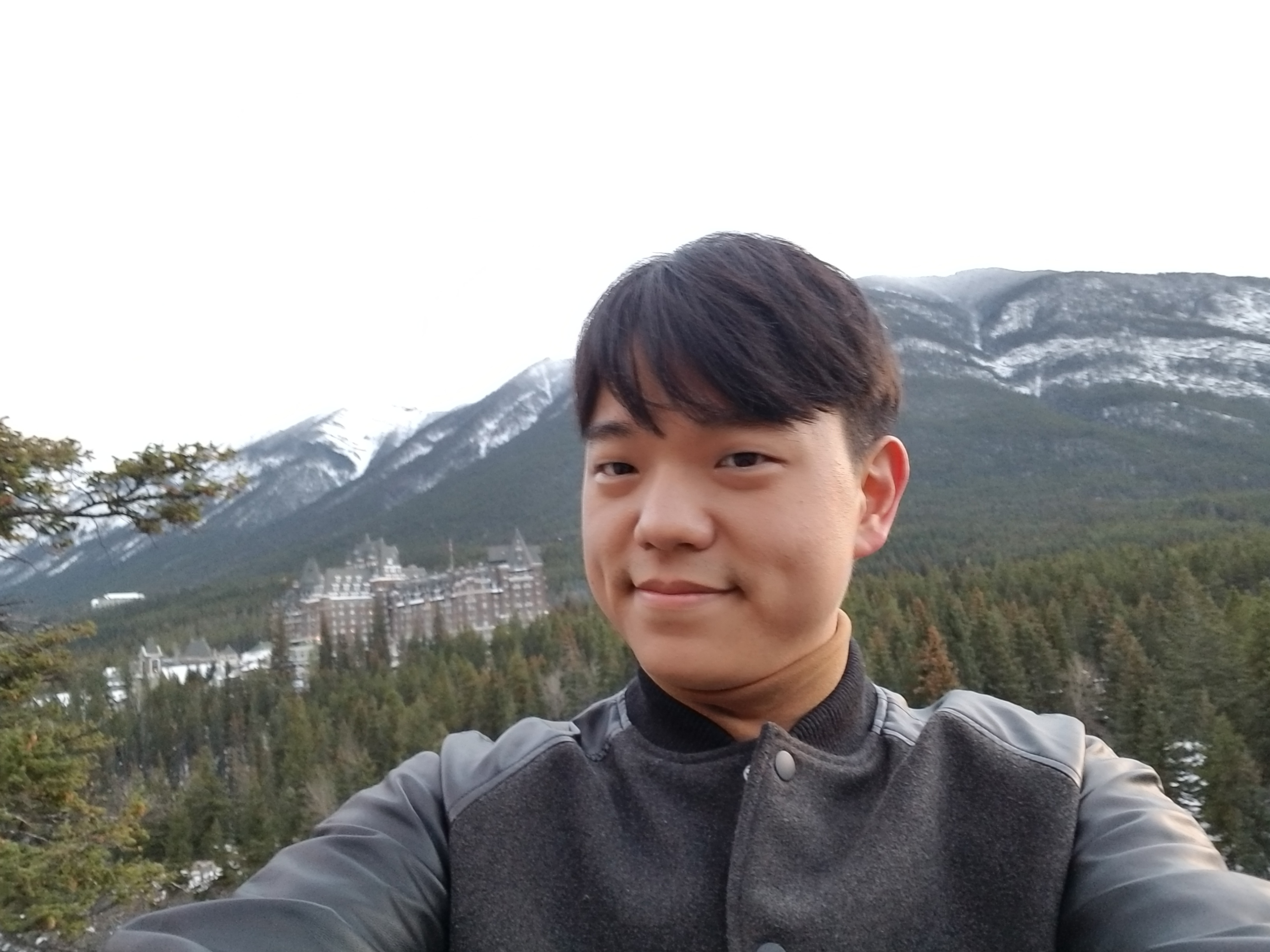# Changho HanPostal address: Department of Mathematics, Harvard University, 1 Oxford Street., Cambridge, MA 02138
Email address: chhan (at) math (dot) harvard (dot) edu
Office: 431d Science Center

About me: I am a fifth year graduate student at Harvard University. Starting on Fall 2019, I will be a Limited Term Assistant Professor at the University of Georgia.

My research is primarily in algebraic geometry. In particular, I am mainly interested in geometric and arithmetic properties of moduli spaces. See my research statement for more details on my mathematical interests.

Here is my CV.

## Teaching

I have taught the following courses at Harvard:

• Math 1b, as a coaching fellow (integration, series and differential equations) which transitions into a teaching fellow, Spring 2019

• Math 1b, as a teaching fellow (integration, series and differential equations), Fall 2017

• Math 21b, as a teaching fellow (linear algebra and differential equations), Spring 2017

• Math 258Y, as a course assistant (graduate topics course on degenerations in algebraic geometry), Fall 2016

• Math 1b, as a teaching fellow (integration, series and differential equations), Fall 2015